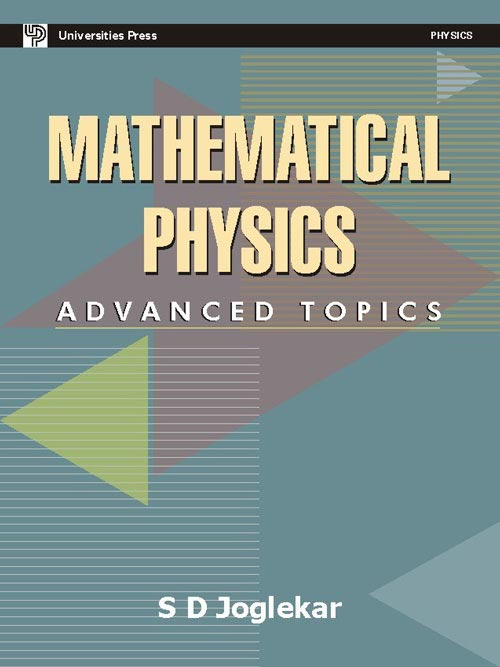#### Price: 695.00

Mathematical Physics: Advanced Topics is the second of the two-volume set on mathematical physics by the author. In volume 1, Mathematical Physics: The Basics, topics such as vectors and tensors, curvilinear coordinates, linear vector spaces and operators, and complex variables and their applications were covered. This book addresses the more advanced topics: partial differential equations, ordinary differential equations, special functions including gamma, beta and Bessel functions, classical orthogonal polynomials and spherical harmonics. It also includes generalized functions, the Dirac-delta function, Fourier transforms and a brief introduction to group theory. A large number of solved problems and exercises have been included in each chapter. Together with Mathematical Physics: The Basics, this book will serve as a comprehensive textbook for M.Sc. and Ph.D. students of physics and chemistry. Engineering students will also find the set a valuable reference tool.

Professor S. D. Joglekar is at the department of physics, Indian Institute of Technology Kanpur, with over 22 years of teaching experience at postgraduate level and over 25 years of research experience in High-Energy Physics. He is a fellow of the Indian National academy of Sciences, Allahabad and Maharashtra Academy of Sciences.

• Preface
• Ordinary Differential Equations
• Gamma (Factorial) and Beta Functions
• Bessel Functions
• Some Special Functions and Spherical Harmonics
• Partial Differential Equations
• Generalized Functions, the Dirac Delta Function and the Fourier Transform
• Group Theory
• Appendix
• References
• Index

`